# Help with Math Homework

Get the math help you need at Bright Hub Education. You’ll find homework help and study tips for all grade levels of K – 12, from basic addition and subtraction guides to advanced algebra and calculus concepts. Whether you are a grade school student looking for multiplication and division techniques, a middle school student struggling with early algebraic concepts, or a high school student studying trigonometry and advanced calculus in preparation for AP or college level math, you’ll find clear, concise and helpful guidance on the math concepts and problems you’ve been struggling with.

## A Guide to Adding Mixed Fractions: Two Techniques

Confused about how adding mixed fractions works? You can add them easily using either one of these step-by-step techniques.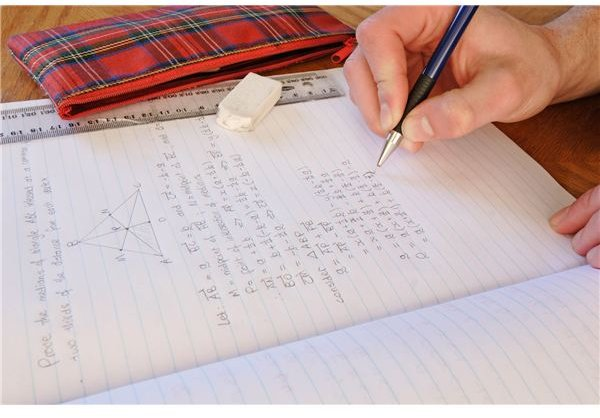## Learn How to Calculate an Average: Steps to Determine Average Rate of Change Plus Two Examples

Once you learn how to calculate an average, you will have mastered one of the most useful and basic statistical calculations available. This article provides example problems and questions on calculating averages to help students master the concept.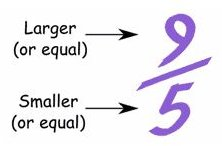## Learn About Improper Fractions and How to Reduce Them

Are you struggling to understand improper fractions and how you can reduce them to the lowest terms? Understanding this is something that is necessary for adding, subtracting, multiplying and dividing fractions, a basic skill which you will need as you continue learning math in the higher grades.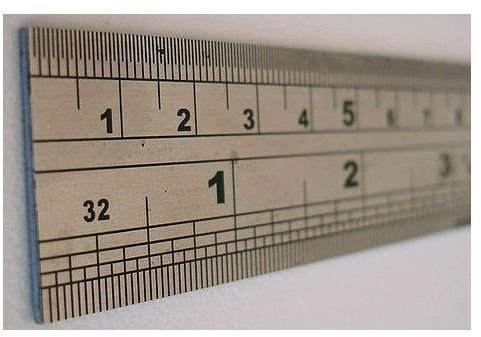## Formulas and Examples of How to Convert Cubic Feet into Cubic Yards

Once you learn how to convert cubic feet into cubic yards, you can learn how to do many other types of conversions. Being able to convert between different units of measurement is an important skill for analysts, architects and other professions. Read on for more homework help!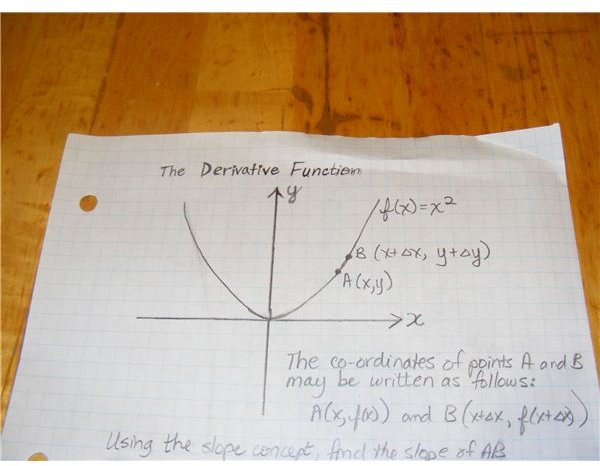## Finding the Derivative of a Function in Calculus

The derivative can be described as the slope, and the limit of a function. The derivative is a function that can be solved. Students can easily solve derivative problems if they know the theory, the rules that apply to solving it and see several examples of finding the derivative in Calculus.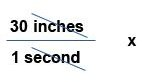## How to Convert Inches per Second to Miles per Hour with Cross Multiplication

Need to know how to convert inches per second to miles per hour? The good news is, the math isn’t actually that hard. Here is how to solve this problem using cross-multiplication, as well as with the conversion factor. Also, you can use these sample problems to practice the conversion.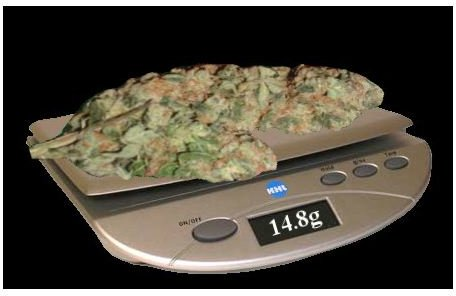## Tricks of Unit Conversion: How to Convert Ounces to Grams, Both Troy & Avoirdupois Ounce

Unit conversion is a mandatory topic to study when in physics. Knowing how to convert ounces to grams is a must for solving problems that deal with objects of constant and varying mass. Discover all the tricks of how to convert ounces to grams in this article.